Type
Solution Manual
Book Title
Fundamentals of Investments: Valuation and Management 8th Edition
ISBN 13
978-1259720697

### 978-1259720697 Chapter 10 Lecture Note

January 2, 2020
Chapter 10
Bond Prices and Yields
Slides
10-1. Chapter 10
10-2. Bond Prices and Yields
10-3. Learning Objectives
10-4. Bond Prices and Yields
10-5. Bond Basics, I.
10-6. Bond Basics, II.
10-7. Straight Bond Prices and Yield to Maturity
10-8. The Bond Pricing Formula
10-9. Example: Using the Bond Pricing Formula
10-10. Example: Calculating the Price of this Straight Bond Using Excel
10-12. Premium, Par, and Discount Bonds, I.
10-13. Premium, Par, and Discount Bonds, II.
10-14. Premium, Par, and Discount Bonds, III.
10-15. Relationships among Yield Measures
10-16. Calculating Yield to Maturity, I.
10-17. Calculating Yield to Maturity, II.
10-19. A Quick Note on Bond Quotations, I.
10-20. A Quick Note on Bond Quotations, II.
10-21. A Quick Note on Bond Quotations, III.
10-22. Callable Bonds
10-23. Yield to Call
10-25. Interest Rate Risk
10-26. Interest Rate Risk and Maturity
10-27. Malkiel’s Theorems, I.
10-28. Malkiel’s Theorems, II.
10-29. Bond Prices and Yields
10-30. Duration
10-31. Example: Using Duration
10-32. Modified Duration
10-33. Calculating Macaulay’s Duration
10-34. Calculating Macaulay’s Duration for Par Bonds
10-35. Calculating Macaulay’s Duration
10-36. Calculating Macaulay’s Duration: Example
10-37. Calculating Duration Using Excel
10-38. Calculating Macaulay and Modified Duration
10-39. Duration Properties
10-40. Properties of Duration
10-41. Bond Risk Measures Based on Duration, I.
10-42. Bond Risk Measures Based on Duration, II.
10-43. Dedicated Portfolios
10-44. Dedicated Portfolios: Scenario
10-45. Dedicated Portfolios: Example
10-46. Dedicated Portfolios: How Does it Work?
10-47. Reinvestment Risk
McGraw-Hill
Education.
Bond Prices and Yields 10-2
10-48. The Cost of Removing Reinvestment Risk Using STRIPS
10-49. Price Risk
10-50. Price Risk versus Reinvestment Rate Risk
10-51. Immunization
10-52. Immunization by Duration Matching
10-53. Immunization by Duration Matching
10-54. Dynamic Immunization
10-55. Useful Internet Sites
10-56. Chapter Review, I.
10-57. Chapter Review, II.
Chapter Organization
10.1 Bond Basics
A. Straight Bonds
B. Coupon Rate and Current Yield
10.2 Straight Bond Prices and Yield to Maturity
A. Straight Bond Prices
C. Relationships Among Yield Measures
D. A Note on Bond Price Quotes
10.3 More on Yields
A. Calculating Yields
B. Yield to Call
C. Using a Financial Calculator
10.4 Interest Rate Risk and Malkiel's Theorems
A. Promised Yield and Realized Yield
B. Interest Rate Risk and Maturity
C. Malkiel's Theorems
10.5 Duration
A. Macaulay Duration
B. Modified Duration
C. Calculating Macaulay Duration
D. Properties of Duration
10.6 Bond Risk Measures Based on Duration
A. Dollar Value of an 01
B. Yield Value of a 32nd
10.7 Dedicated Portfolios and Reinvestment Risk
A. Dedicated Portfolios
B. Reinvestment Risk
10.8 Immunization
A. Price Risk versus Reinvestment Rate Risk
B. Immunization by Duration Matching
C. Dynamic Immunization
10.9 Summary and Conclusions
McGraw-Hill
Education.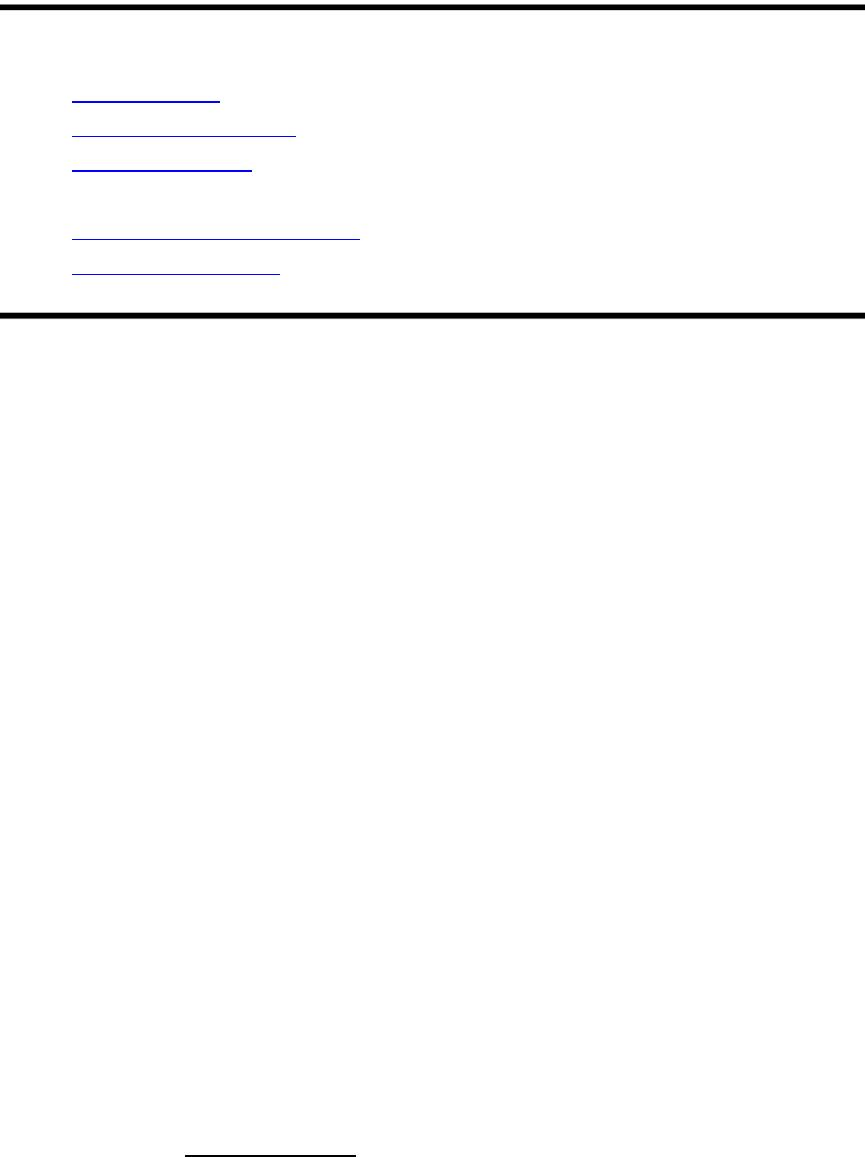Bond Prices and Yields 10-3
Selected Web Sites
www.sifma.org (check out the bonds section)
www.zionsdirect.com (search for bonds)
www.gobaker.com (a practical view of bond portfolio management)
finra-markets.morningstar.com (bond basics and current market data)
www.investinginbonds.com (more on bond prices and yields)
www.bloomberg.com (for information on government bonds)
Annotated Chapter Outline
10.1 Bond Basics
A bond is a security that offers a series of fixed interest payments, and a fixed
principal payment at maturity. Maturities typically range from 2 years to 30 years,
with some from 50 to 100 years. There are even a few perpetuities (consols).
A. Straight Bonds
The most common bond is the straight bond, an IOU that obligates the issuer to
pay to the bondholder a fixed sum of money at the bond's maturity along with
constant, periodic interest payments. Most bonds pay interest semiannually, with
a face value of \$1,000 per bond. Different types of bonds and bond features will
be discussed in the next few chapters.
B. Coupon Rate and Current Yield
Coupon rate: This is a bond's annual coupon divided by its price. It is also
called coupon yield or nominal yield.
Current yield: A bond's annual coupon divided by its market price.
A bond's coupon rate is expressed as a percentage of face value:
McGraw-Hill
Education.
Coupon rate =Annual coupon
Par valueBond Prices and Yields 10-4
A bond's current yield is inversely related to its price and is calculated as follows:
10.2 Straight Bond Prices and Yield to Maturity
Yield to maturity (YTM): This is the discount rate that equates a bond's
price with the present value of its future cash flows. It is also called
promised yield, or just yield.
Yield to maturity is the most important yield measure for a bond. If just the term
"yield" is used it means yield to maturity.
A. Straight Bond Prices
To calculate the price of a bond:
where C = annual coupon (sum of the two semiannual coupons), FV = face
value, M = maturity in years, and YTM = yield to maturity.
The bond price is based on two components: the present value of the annuity of
the interest payment cash flows and the present value of the face value received
at maturity. Bond prices can be easily calculated using a financial calculator or
The three price descriptions, based on the selling price of the bond, are:
Premium bonds: price is greater than par value and YTM is less than the
coupon rate.
Discount bonds: price is less than par value and YTM is greater than the
coupon rate.
Par bonds: price is equal to par value and YTM is equal to the coupon
rate.
McGraw-Hill
Education.
Current yield =Annual coupon
Bond price
Bond Price =C
YTM
[
11
(
1+YTM
2
)
2M
]
+FV
(
1+YTM
2
)
2M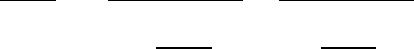Bond Prices and Yields 10-5
Figure 10.1 shows that, with no changes in YTM, the price of a premium bond
will decrease and the price of a discount bond will increase as the bond
approaches maturity. It also shows that the longer the term to maturity, the
greater the premium or discount on a bond.
C. Relationships Among Yield Measures
Taken together, the bond yield measures result in:
Premium bonds: Coupon rate > current yield > YTM
Discount bonds: Coupon rate < current yield < YTM
Par value bonds: Coupon rate = current yield = YTM
D. A Note on Bond Price Quotes
If you buy a bond between coupon dates, you will receive the next coupon
payment (and might have to pay taxes on it). However, when you buy the bond
between coupon payments, you must compensate the seller for any accrued
interest.
The convention in bond price quotes is to ignore accrued interest. This results in
what is commonly called a clean price (i.e., a quoted price net of accrued
interest). Sometimes, this price is also known as a flat price.
The price the buyer actually pays is called the dirty price, because accrued
interest is added to the clean price. The price the buyer actually pays is
sometimes known as the full price, or invoice price.
10.3 More on Yields
A. Calculating Yields
To calculate a bond's yield to maturity, we use the same formula as for the bond
price:
This calculation is computed on a trial-and-error basis, unless a financial
McGraw-Hill
Education.
Bond Price =C
YTM
[
11
(
1+YTM
2
)
2M
]
+FV
(
1+YTM
2
)
2M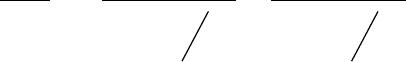Bond Prices and Yields 10-6
Lecture Note: Texas Instruments offers an “emulator” for their BAII-Plus
calculator. This software is used to project a workable image of the calculator.
This is helpful for showing students how to perform various calculations.
For yields, it is important to note that the YTM is an expectation. Actually earning
the YTM requires the bond to be held until maturity (or interest rates remain
constant) and coupons be reinvested at the YTM.
B. Yield to Call
Callable bond: A bond is callable if the issuer can buy it back before it
matures.
Call price: The price the issuer of a callable bond must pay to buy it back.
Call protection period: The period during which a callable bond cannot
be called is the call protection period. It is also called a call deferment
period.
Yield to call (YTC): Measure of return that assumes a bond will be
redeemed at the earliest call date.
If interest rates decrease it is likely a callable bond will be called. Therefore, the
yield to call becomes the relevant measure of the bond's yield. Its calculation is
similar to the bond's YTM:
Where C = constant annual coupon, CP = call price of the bond, T = time in years
until earliest possible call date, and YTC = yield to call. If the bond is callable at
par, then for a premium bond the YTM is greater than the YTC, and for a discount
bond the YTM is less than the YTC.
C. Using a Financial Calculator
Understanding a financial calculator is a necessity. This section provides a
screenshot of one of the most common financial calculators – the Texas
Instruments BAII-Plus.
With regard to bonds, the time value buttons are the key: N (number of periods),
I/Y (periodic interest rate), PV (price), PMT (periodic coupon), and FV (face).
10.4 Interest Rate Risk and Malkiel's Theorems
McGraw-Hill
Education.
Callable Bond Price =C
YTC
[
11
(
1+YTC
2
)
2T
]
+CP
(
1+YTC
2
)
2T
Bond Prices and Yields 10-7
Interest rate risk: The possibility that changes in interest rates will result
in losses in a bond's value.
A. Promised Yield and Realized Yield
Realized yield: The yield actually earned or "realized" on a bond.
A bond's realized yield will only equal its promised yield if interest rates don't
change over the life of the bond. This may be due to two factors. First, if you sell
the bond before maturity, the bond may be selling at a premium or a discount due
to changing interest rates. Second, to achieve the promised yield, all coupons
have to be reinvested at that yield, an unlikely event.
B. Interest Rate Risk and Maturity
Notice from Figure 10.3 that decreasing yields cause bond prices to rise, but
long-term bonds increase more than short-term. Similarly, when yield increases,
long-term bonds decrease more in price than short-term bonds.
C. Malkiel's Theorems
Malkiel's theorems summarize the relationship between bond prices, yields,
coupons, and maturity. Malkiel’s Theorems are paraphrased below (see text for
exact wording). Note, all theorems are ceteris paribus:
Bond prices move inversely with interest rates.
The longer the maturity of a bond, the more sensitive is its price to a
change in interest rates.
The price sensitivity of any bond increases with its maturity, but the
increase occurs at a decreasing rate.
The lower the coupon rate on a bond, the more sensitive is its price to a
change in interest rates.
For a given bond, the volatility of a bond is not symmetrical, i.e., a
decrease in interest rates raises bond prices more than a corresponding
increase in interest rates lowers prices.
10.5 Duration
Duration: A widely used measure of a bond's sensitivity to changes in
bond yields.
McGraw-Hill
Education.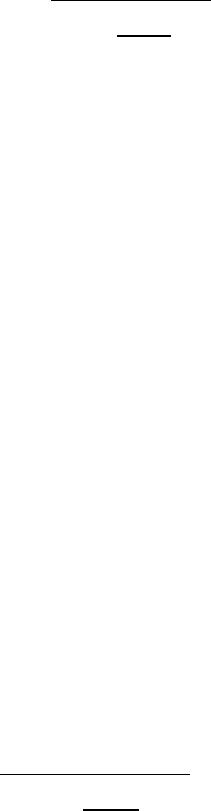Bond Prices and Yields 10-8
Lecture Tip: To give students a feel for duration, it is helpful to give them some
alternative explanations of duration. The following are some examples:
Measures the combined effect of maturity, coupon rate, and YTM on the
bond’s price sensitivity,
Measure of the bond’s effective maturity,
Measure of the average life of the security, and
Weighted average maturity of the bond’s cash flows.
A. Macaulay Duration
Macaulay's duration, commonly called duration, allows the investor to calculate
the approximate change in price with respect to changes in yields:
Example: Suppose a bond has a Macaulay duration of 6 years, and a current
yield to maturity of 10%. If the yield to maturity declines to 9.75%, the resulting
percentage change in the price of the bond is:
Pct Change in Bond Price = -6 X [(.0975 – .10) / (1 + .10/2)] = -1.4286%
Two bonds with the same duration, but not necessarily the same maturity, have
about the same price sensitivity to a change in yields. Note, however, that this
approximation only holds for small changes in yields.
Big changes in yields require a more advanced correction, called a convexity
various yields. You will see that a curved equation results. When yield changes a
little bit, the duration equation estimates the price change with a linear
approximation. Because duration is a linear approximation (i.e., a derivative), its
error will increase with large changes in yields. That is, the duration formula
makes bigger errors in predicting bond price percentage changes when big yield
B. Modified Duration
To calculate modified duration from Macaulay duration:
McGraw-Hill
Education.
% Change in Bond Price ≃−Duration ×Change in YTM
(
1+YTM
2
)
Modified Duration =Macaulay Duration
(
1+YTM
2
)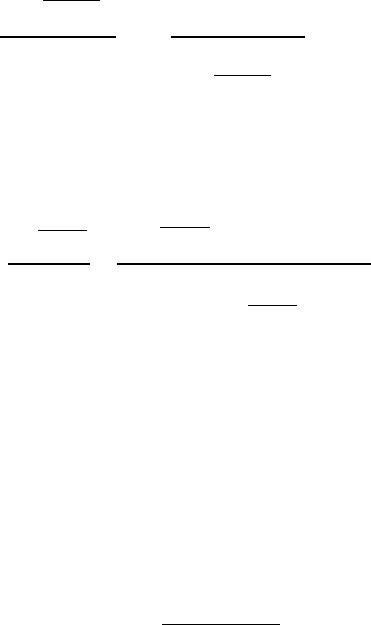Bond Prices and Yields 10-9
To calculate the approximate percentage price change in bond price with respect
to a change in yields using modified duration:
That is, to calculate the percentage change in the bond’s price, we just multiply
the modified duration by the change in yields.
C. Calculating Macaulay Duration
To calculate Macaulay duration for a par value bond:
To calculate Macaulay's duration for any bond:
Lecture Tip: Students sometimes take a step back when asked to calculate
duration, so it is important to remind them that the reason that duration is
calculated is because we want to see how much the price of a bond will change
given a change in interest rates (i.e., yields). Otherwise, the formulas may seem
to be just a “plug and chug” exercise to them. It also helps to present this
alternative formula, which is much simpler in form and easier to explain, but no
easier to calculate. This formula relates to the calculation in Table 10.1 in the text.
D. Properties of Duration
Briefly summarizing the properties of duration:
Longer maturity, longer duration,
Duration increases at a decreasing rate,
Higher coupon, shorter duration, and
McGraw-Hill
Education.
% Change in bond price Modified Duration ×Change in YTM
Par Value Bond Duration =
(
1+YTM
2
)
YTM
[
11
(
1+YTM
2
)
2M
]
MD =
1+YTM
2
YTM
(
1+YTM
2
)
+M
(
CYTM
)
YTM +C
[
(
1+YTM
2
)
2M 1
]
Macaulay Duration=
t=1
nPV
(
CFt
)
Bond Price ×t
Bond Prices and Yields 10-10
Higher yield, shorter duration.
It is also useful to remember the following property that is related to duration:
Zero coupon bond: duration = maturity
10.6 Bond Risk Measures Based on Duration
A. Dollar Value of an 01
A popular measure of interest rate risk among bond professionals is the dollar
value of an 01. The dollar value of an 01 measures the change in bond price
resulting from a one basis point change in yield to maturity.
The dollar value of an 01 is also known as the value of a basis point. The
dollar value of an 01 can be approximated using the modified duration of a bond
as follows:
Dollar value of an 01 ≈ −Modified duration × Bond price × .01
B. Yield Value of a 32nd
When bond prices are quoted in 1/32’s of a point, as they are, for example, with
U.S. Treasury notes and bonds, the yield value of a 32nd is often used by bond
professionals as an additional or alternative measure of interest rate risk.
The yield value of a 32nd is the change in yield to maturity that would lead to a
1/32 change in bond price. A simple approximation of the yield value of a 32nd is
to multiply the dollar value of an 01 by 32 and then invert the result:
Yield value of a 32nd ≈ 1 / (32 × Dollar value of an 01)
10.7 Dedicated Portfolios and Reinvestment Risk
A. Dedicated Portfolios
Dedicated portfolio: A bond portfolio created to prepare for a future cash
outlay.
When setting up a dedicated portfolio the investment manger must recognized
that the portfolio's target date must match the bond portfolio's duration. If the
portfolio is matched based on maturity, yield fluctuations will cause the value of
the portfolio to differ from the future liability payment that is to be secured by the
dedicated portfolio.
McGraw-Hill
Education.
Bond Prices and Yields 10-11
B. Reinvestment Risk
Reinvestment rate risk: The uncertainty about a future or target date
portfolio value that results from the need to reinvest bond coupons at
As market yields change, the reinvestment rate on bond coupons received also
varies. The reinvestment rate risk is the uncertainty about the future value of the
portfolio due to its coupons being reinvested at unknown future interest rates.
10.8 Immunization
Immunization: Constructing a portfolio to minimize the uncertainty
surrounding its target date value.
A. Price Risk versus Reinvestment Rate Risk
Price risk: The risk that bond prices will decrease, which arises in
dedicated portfolios when the target date value of a bond or bond portfolio
is not known with certainty.
For a dedicated portfolio, increasing interest rates decrease bond prices (price
risk), but increase the future value of reinvested coupons (reinvestment rate risk).
If interest rates decrease, the reverse occurs. The key observation is that price
risk and reinvestment rate risk offset each other.
B. Immunization by Duration Matching
The key to immunizing a dedicated portfolio is to match its duration to its target
date. Immunization is often referred to as duration matching. This causes the
impacts of price risk and reinvestment rate risk to offset each other and maintain
the target date value of the portfolio, even though interest rates may fluctuate.
C. Dynamic Immunization
Dynamic immunization: Periodic rebalancing of a dedicated bond
portfolio to maintain a duration that matches the target maturity date.
When a portfolio is immunized, it is essentially protected against one interest rate
change. As interest rates fluctuate with time, the duration of the portfolio will also
change. Therefore, a dedicated bond portfolio should be rebalanced periodically
to maintain a portfolio duration that is matched to the target date. This is called